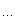# coreBugZJ

## reverse order 1

Time Limit: 1 Sec Memory Limit: 128 MB
Submissions: 531 Solved: 87

## Description

Here is a sequence a1..n, which is a disordered sequence from 1 to N. If i < j and ai > aj, then <i, j> is called a pair of inversion. And b1..n-1 is defined as follows, bk is the number of the total inversion pairs in array a, when i<=k<j. Now the array b is required while the array a is known.

## Input

Several cases end with the end of the file;

And each of the cases includes two lines, a integer n(2<=n<=10^5)in the first line, and the second line followed with n integer, which is in the presentation of array a;

## Output

Output the answer of each case in a line, namely the array b, and a space is required between the adjacent integers.

```5
3 1 4 2 5```

## Sample Output

`2 1 2 0`
` `
`树状数组。。。`
``` 1#include <iostream> 23using namespace std; 45typedef  long long  Lint; 67const int L = 200009; 89int n, a[ L ];10Lint b[ L ], al[ L ], sl[ L ], ar[ L ], sr[ L ];1112void init( Lint a[], Lint s[] ){13int i;14for ( i = 0; i <= n; ++i ){15a[ i ] = s[ i ] = 0;16}17}1819#define  getBit(i)  (i&(i^(i-1)))2021void add( Lint a[], Lint s[], int i, Lint d ){22a[ i ] += d;23while ( i <= n ){24s[ i ] += d;25i += getBit(i);26}27}2829Lint get( Lint a[], Lint s[], int i ){30Lint res = -a[ i ];31while ( i > 0 ){32res += s[ i ];33i -= getBit( i );34}35return res;36}3738int main(){39int i;40while ( cin >> n ){41for ( i = 1; i <= n; ++i ){42cin >> a[ i ];43}44init( al, sl );45init( ar, sr );46for ( i = n; i > 0; --i ){47add( ar, sr, a[ i ], 1 );48}49b[ 1 ] = get( ar, sr, a[ 1 ] );50add( al, sl, n+1-a, 1 );51for ( i = 2; i < n; ++i ){52add( ar, sr, a[i-1], -1 );53add( al, sl, n+1-a[i], 1 );54b[ i ] = b[ i - 1 ] - get( al, sl, n+1-a[i] ) + get( ar, sr, a[i] );55}56cout << b[ 1 ];57for ( i = 2; i < n; ++i ){58cout << " " << b[ i ];59}60cout << "\n";61}62return 0;63}64```

posted on 2011-04-09 18:35 coreBugZJ 阅读(818) 评论(0)  编辑 收藏 引用 所属分类: ACM